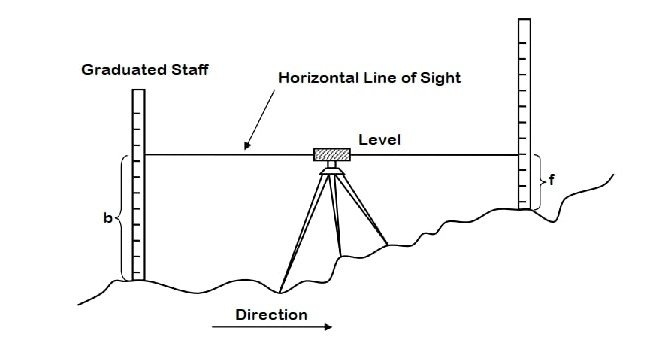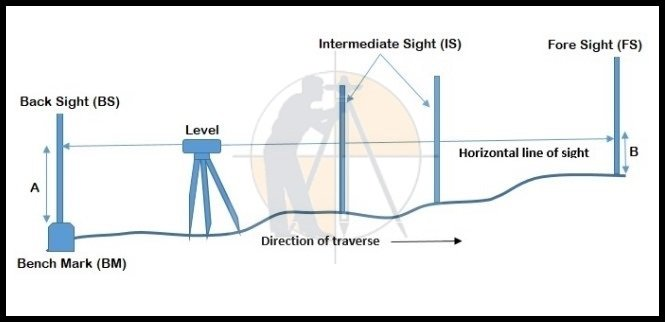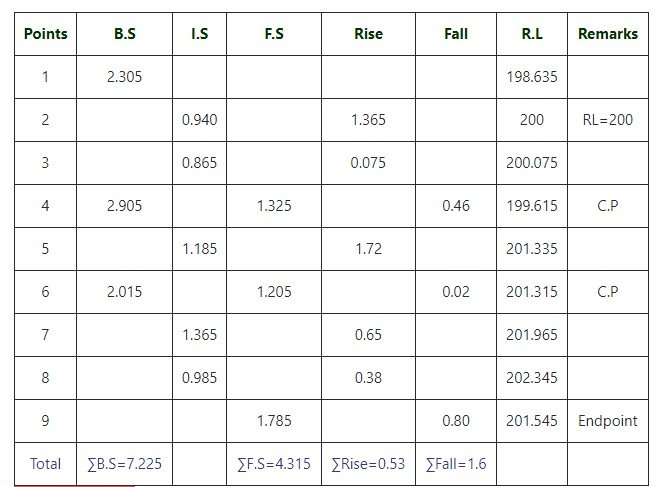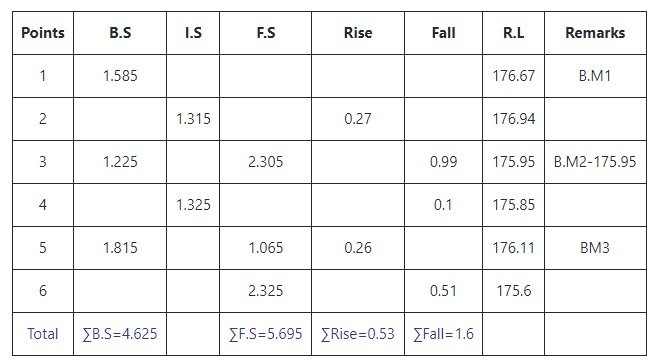Breaking News
Home / Land Surveying / Rise and fall method with Examples

# Rise and fall method with Examples

### Rise and fall method with Examples.

In This Article i will discuss About the topic of Rise and Fall Method with Complete Detail.

Rise & fall is the method of Land Surveying to solve the levelling to find out difference in elevation & elevation of 2 points. In this methodLike we need to calculate the difference in elevation of the staff of 2 points. The 2 stations points where the staff readings are taken, we know the RL of the ground of 1 station & we have to find the RL of another.

### What is the Back Sight?

Back Sight is the first reading of a staff (levelling rod) which remains unchanged when the levelling apparatus is taken to another or a new point after the levelling instrument is set up & levelled on the first point.  And simply it can be defined as the backward reading of previous station point. It is the taken on known reduced level or benchmark.

### What is the Inter sight?

All the reading which is taken between back sight & the foresight is called inter-sight.### What is the Fore Sight?

Foresight is a reading of the staff or levelling rod which is taken in the forward direction of leveling process or the staff reading which is to be determined & it is the last reading of the whole surveying process. It is considered as negative & deducted from Height of the Instrument to determine RL of the point.

### What is the Benchmark?

Benchmark is considered as the fixed point of known elevation point through which is the reduced level of the other point is determined.

It is arbitrary or permanent reading through which the surveying works are done & calculation of the reduced level is done. For GTS surveys work, the Land surveyor uses an identified or permanent benchmark to calculate elevation of the points.

### Rise & Fall Method Formula.

Determine rise or fall using.

B.S – I.S or I.S – F.S

Where,

1. B.S= back sight
2. F.S= fore sight
3. I.S= intermediate sight

If,

B.S-F.S=+ve (Rise) and B.S-F.S=-ve (Fall)

### Determining new R.L.

New R.L= Old R.L – fall = Old R.L + rise

### Arithmetic checking

∑B.S – ∑F.S = ∑Rise – ∑Fall = Last R.L – First R.L

### Advantages of the Rise & Fall Method

1. The advantage of the Rise & fall method is that it gives more accuracy in the reading & it is includes many steps.
2. This method is quite complicated & is not easy to use.
3. It is used where the change point is more & the reduced level takes the more time. Imagination or visualization is required for the nature of the ground.
4. The complete check is done for all the readings.

Let us discuss this with few real calculations related to the Rise & Fall method.

### Example 1.

The following staff readings were obtained during the leveling work with the instrument being shifted after the 4th, 7th & 10th. Readings:  2.305, 0.940, 0.865, 1.325, 2.905, 1.185, 1.205, 2.015, 1.365, 0.985 & 1.785. Find reduced levels of the remaining points if RL of the second turning point is 200.00### Arithmetic Check.

∑B.S –∑F.S = ∑Rise – ∑Fall = Last R.L – First R.L

= 7.225 – 4.315 =4.19 – 1.28 = 201.545 – 198.635

=  2.91 = 2.91 = 2.91

Hence checked.

### Example 2.

Eight readings were taken with the level in sequence as follows: 1.585, 1.315, 2.305, 1.225, 1.325, 1.065, 1.815, & 2.325. The level was shifted after the third & sixth readings. The second change point was the benchmark of elevation 175.975. Find the reduced levels of the remaining stations. Use rise and fall method.### Arithmetic Check.

∑B.S –∑F.S = ∑Rise – ∑Fall = Last R.L – First R.L

= 4.625 – 5.695= 0.53-1.6 = 175.6- 176.67

= -1.07 = -1.07 = -1.07

Hence checked.

Raja Junaid Iqbal

### THANKS.